## 19 Mar 2016

### Priest, Ch10 of Logic: A Very Short Introduction, “Vagueness: How Do You Stop Sliding Down a Slippery Slope?”, summary

[
Search Blog Here. Index-tags are found on the bottom of the left column.]

[Central Entry Directory]
[Logic & Semantics, Entry Directory]
[Graham Priest, entry directory]
[Priest’s Logic: A Very Short Introduction, entry directory]

[Bracketed commentary and boldface are my own. Please forgive my typos, as proofreading is incomplete. I am not trained in logic, so at times my summaries may be unhelpful or misleading. Please consult and trust the original text, which is absolutely wonderful.]

Summary of

Graham Priest

Logic: A Very Short Introduction

Ch.10
Vagueness: How Do You Stop Sliding Down a Slippery Slope?

Brief Summary:
A thing can change gradually over time. A true statement about that thing’s status at the beginning can later be false at the end of the development. But in many cases, it is not clear when exactly during that development the status changes without ambiguity. “Jack is a child” is true when Jack is very young and not true when Jack is old; but, when precisely in his young adult years does it cease being entirely true and instead “Jack is an adult” becomes entirely true? This issue is related to sorites paradoxes. Consider that “Jack is a child” is true at the beginning, and “If Jack is a child at the beginning, then he is still a child one second later” also is probably also true. That means by modus ponens, “Jack is a child one second later” is true. Using this same sort of reasoning, we can then conclude that Jack is a child two seconds later, and so on, meaning that he never ceases being a child. (We reiterate the structure, taking the affirmed prior conclusion that Jack is still a child in the  succeeding second, and use it as a premise in an argument of the same structure, allowing us to conclude he is a child in yet the next succeeding second, and so on infinitely).  One solution to these issues is to use fuzzy truth values. We can say for example that when he is 3 years old, the statement “Jack is a child” has a full truth value of 1. At 9 years “Jack is  child” has a truth value of 0.75. At 14 years, 0.5. At 19 years, 0.25. And at 24 years, 0. And when we apply truth functional operators to statements with  values between 1 and 0, we can determine the different resulting fuzzy values. Also, we can say that an inference is valid when both the conclusion and the premises meet a certain minimum level of truth value, which is determined by the actual context to which the statements apply. What we find then is that the sorites paradox does not hold when we use this fuzzy system. [For, in order for the modus ponens inference to work in all steps, we will need the minimum value to be 0 (in order to accommodate the final transitional step), which is too low to be meaningful.] Also, fuzzy values do not clear up the situation entirely, because we have the same problem when we need to determine precisely at what point the values change from 1 to something less than 1.

Summary

[We previously discussed identity, and we noted that something’s properties can change, which then raises the difficulty of determining whether or not we can say that the thing’s identity has changed.] Priest will discuss another problem with identity; in this case it is the problem of vagueness. He poses a very interesting thought experiment to illustrate the issue. What if we replace something’s parts one by one until it has all new parts. For certain things like machines, we would seem to still have the same identical machine even after all its parts were gradually replaced. [Or at least, it would be very hard to determine at which point its identity changed. If we say it changed with the first replacement, what if that replacement were negligible? And on the atomic level, are there  not such changes happening always anyway? Or, consider if we say it happened with the most final replacement. But also, could not that final replacement be negligible as well? Yet, if we choose some replacement happening between, what would be our criteria for saying it was one middle replacement and not the one coming right before or after it? Priest’s motorcycle example is especially effective, and it makes the issue even more complicated. What if, along with the gradual replacements, we kept the parts and reconstructed the original machine? We would on the  one hand have the replacement machine, which we said could be the same as the original, and we then have the machine made of all the replaced parts, which would also seem to be the original. But how can both be identical to the original machine?]

While we are on the subject of identity, here is another problem about it. Everything wears out in time. Sometimes, parts get replaced. Motor bikes and cars get new clutches; houses get new roofs; and even the individual cells in people’s bodies are replaced over time. Changes like this do not affect the identity of the object in question. When I replace the clutch on my bike, it remains the same bike. Now suppose that over a period of a few years, I replace every part of the bike, Black Thunder. Being a careful fellow, I keep all the old parts. When everything has been replaced, I put all the old parts back together to recreate the original bike. But I started off with Black Thunder; and changing one part on a bike does not affect its identity: it is still the same bike. So at each replacement, the machine is still Black Thunder; until, at the end, it is – Black Thunder. But we know that that can't be right. Black Thunder now stands next to it in the garage.
(70)

Priest gives another example. If a minute incremental change does  not change a basic state of something, then how do we know when there are enough such tiny changes to constitute the change in state? His example of seconds added to childhood illustrates this very well.

Here is another example of the same problem. A person who is 5 years old is a (biological) child. If someone is a child, they are still a child one second later. In which case, they are still a child one second after that, and one second after that, and one second after that, ... So after 630,72o,ooo seconds, they are still a child. But then they are 25 years old!
(70)

[Note: page 71 is a full page figure of the motor cycle situation.]

[Recall from chapter 5 that Eubulides is credited for inventing the liar paradox.] Such arguments as these are now called sorites paradoxes, and they are thought to have been invented by Eubulides. Priest explains where the name comes from: “A standard form of the argument is to the effect that by adding one grain of sand at a time, one can never form a heap; ‘sorites’ comes from ‘soros’, the Greek for heap” (72). He explains that we encounter sorites paradoxes “when the predicate employed (‘is Black Thunder’, ‘is a child’) is vague, in a certain sense; that is, when its applicability is tolerant with respect to very small changes: if it applies to an object, then a very small change in the object will not alter this fact” (72). He notes that in fact, very many of the predicates in our everyday usage are vague. For example, ‘is red’ is vague [because something’s redness can vary slightly to the point of no longer being red], as is ‘is awake’ [perhaps because we “drift-off” to sleep and at times awaken gradually]. He even says that ‘is dead’ is a vague predicate, because it takes time to die [I am not entirely sure I understand this one. It seems the idea is that because we taper-off into death, there is no way to know exactly at what point in that passing-away is the point of death. So imagine the instant before all life is gone. There would seem to be too little life left to really say the person is alive. But I am not sure.] Thus such “slippery slope” sorties arguments “are potentially endemic in our reasoning” (72).

Priest will now present a symbolic formulation of the paradox with the childhood example illustrating it. We have a five year old child whom we will call ‘Jack’, and we will consider Jack after certain  numbers of seconds have passed. We will write ‘Jack is a child after 0 seconds’ as a0. And thus, a1 will mean ‘Jack is a child after 1 second,” and so on for each subscript numeral. We can then think more generally of the formulation using the variable n, and say that an means “Jack is a child after n seconds,” when n stands for some number value. We will pick an unspecified very large number that is “at least as great as 630,720,000,” and we will just call it k. [So in the diagram below, ak-1 would mean, the second right before this very large number.] We will then  make a series of inferences using modus ponens. [He discusses modus ponens in chapter 6 and chapter 7.] We first note that Jack is still a child at five years old, that is, after zero seconds have passed. So we first assert a0, which states this fact. We then formulate a conditional, “If Jack is a child after 0 seconds, then he is a child after 1 second”, or: a0 a1. Now, since we already asserted a0 , then by modus ponens, we can infer a1. We then take as a premise in the next inference, where we, following the same reasoning, say that if Jack is a child after 1 seconds, then he is a child after 2 seconds.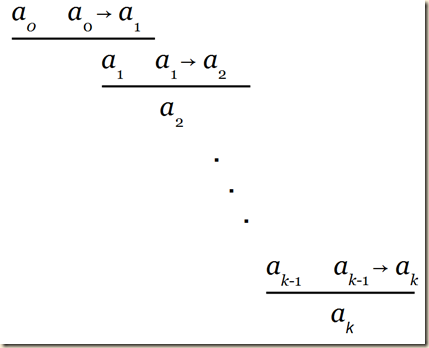As we noted, ak means 630,720,000 seconds later Jack is still a child, which we know to be false. “Something has gone wrong, and there doesn’t seem much scope to manoeuvre” (72).

Priest then notes one solution using fuzzy logic. The idea seems to be that since in reality one gradually changes from childhood to adult through a series of degrees of variation, then there are degrees of truthfulness to the claim that “Jack is a child” at different point along his growth.

So what are we to say? Here is one answer, which is sometimes called fuzzy logic. Being a child seems to fade out, gradually, just as being a (biological) adult seems to fade in gradually. It seems natural to suppose that the truth value of ‘jack is a child’ also fades from true to false. Truth, then, comes by degrees. Suppose we measure these degrees by numbers between 1 and o, 1 being complete truth, o complete falsity. Every situation, then, assigns each basic sentence such a number.
(73)

[The next idea seems to be that we will want to incorporate operators to sentences with fuzzy truth values, and the way to do that for negation is to give the negated term the value that the non-negated term lacks in comparison to the full 1 value.]

What about sentences containing operators like negation and conjunction? As Jack gets older, the truth value of  ‘Jack is a child’ goes down. The truth value of ‘Jack is not a child’ would seem to go up correspondingly. This suggests that the truth value of ¬a is 1 minus the truth value of a. Suppose we write the truth value of a as |a|; then we have:

a | = 1 – |a|

Here is a table of some sample values: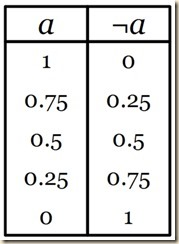(73)

[Priest then will show how we calculate the truth value for a conjunction of sentences with fuzzy values. The reasoning here is less obvious I think. One element of the thinking seems to be that by conjoining such sentences, we are not adding all their truth values together nor are we finding the average. So let us suppose that in addition to “Jack is a child” we also assume that Jack is training to become a cook, and so we also have “Jack is a cook”. Suppose also that Jack is halfway to becoming an adult and halfway to becoming a cook. When we conjoin those statements, “Jack is a child and Jack is a cook”, the value should still be 0.5, because that represents his situation on both accounts. However, suppose that Jack is halfway to becoming an adult and only a quarter of the way to becoming a cook. For some reason, in this case, the conjunction “Jack is a child and Jack is a cook” takes the value of 0.25. I am not sure why. For instance, why not the average of the two values? One possible thing to consider is that the normal truth evaluation for conjunction seems to have a similar sort of pattern. If even one conjunct is false, then the whole thing is false. So the value of the whole can be no “greater” than the value of the “lowest” term. But I am not sure if that helps us understand why in the fuzzy value assessment of conjunction, we always take the lowest value as being the value for the whole conjunction.]

What about the truth value of conjunctions? A conjunction can only be as good as its worst bit. So it’s natural to suppose that the truth value of a & b is the minimum (lesser) of |a| and |b|:

|a & b| = Min(|a|, |b|)

|

Here is a table of some values. Values of a are down the left hand column; values of b are along the top row. The corresponding values of a & b are where the appropriate row and column met. For example, if we want to find |a & b|, where |a| = 0.25 and |b| = 0.5, we see where the italicized row and column meet. The result is in boldface.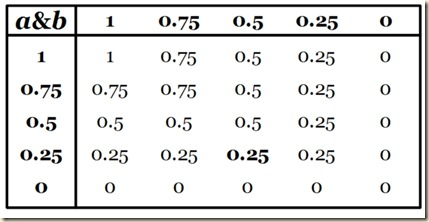(73-74)

[For disjunction, we go with the value of the greater disjunct. I am not sure why, again. But using the reasoning we had before, it could be because normally a disjunction is true so long as at least one disjunct is true. And it is false only if both terms are false. So perhaps the idea is that we always go with the highest value in the two-value system, so we likewise go with the highest value in the fuzzy value system.]

Similarly, the value of a disjunction is the maximum (greater) of the values of the disjuncts:

|a b| = Max(|a|, |b|)

I leave it to you to construct a table of some sample values.

(p.74)

[I am not sure I have it right, but below is possibly an option.]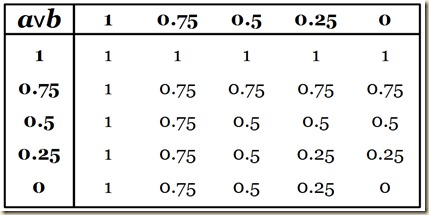Priest  notes that ¬, &, ∨ are truth functional in fuzzy logic, because the truth value when these operators are applied is determined on the basis of the truth values of the original terms. [We discussed truth functions in chapter 2. What makes operators truth functional is if the output values are computable on the basis of the input values. The only difference now is that the values that we input and receive as output are between 0 and 1 rather than being T or F.] This is apparent when we compare the truth tables for the two systems. (74). [Below we see that clearly with negation (just ignore the fuzzy values in the middle).

With conjunction, look just at the places where 1 and 0 intersect in the fuzzy table. You see that it is only where 1 and 1 intersect that the output value is 1, just like how the time the output value is T is where both conjuncts are T.

And look again at the fuzzy disjunction for where 1 and 0 intersect, and you will see it corresponds with the T and F of the other table.

]

Priest now addresses the question of the conditional, →, which we will consider here as truth functional. It is not obvious how we compute fuzzy values for the conditional. But he gives a standard solution, “which at least seems to give the right sorts of results”.

If |a| ≤ |b|: |a→b| = 1
If |b| < |a|: |a→b| = 1 – (|a| – |b|)

(< means ‘is less than’; ≤ means ‘is less than or equal to’.) Thus, if the antecedent is less true than the consequent, the conditional is completely true. If the antecedent is more true than the consequent, then the conditional is less than the maximal truth by the difference between their values. Here is a table of some sample values. (Recall that the values of a are down the left-hand column and those of b are along the top row.)
(75)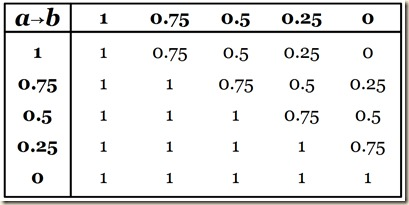[I do not understand why the values are the way they are.  Let us take an example of a conditional from chapter 6: “If she works out regularly then she is fit.” If the truth value of a is 1 (she indeed works out regularly) and b is 0.25 (she is only a little fit) then the whole conditional’s value is 0.25 (it is only a little true that if she works out regularly she will be fit). This makes sense, because she worked out so she should be very fit, but in fact it is only a little so. Now, if a is 0.25 (she only works out a little), and b  is 1 (she is fit), then the whole conjunction is completely true, 1. I am not sure why. Perhaps the idea is that this situation demonstrates that even a little bit of exercise is already enough to result in fitness. This holds even if she is only 0.25 fit. But if it is 0.25 true that she exercised but she is not fit at all, then the whole conditional is 0.75. So she exercised a little, but she is not fit, and for some reason the whole value of “if she works out she will be fit” is 0.75. This perhaps makes sense because she did not really fulfill the conditions enough to qualify entirely. But it is hard to make a general statement about how it all works. It seems one thing we can say is that the conditional on a whole cannot be less true than the consequent, but it can be less true than the antecedent. And any time that the antecedent is at least as true as the consequent, the whole conditional is completely true (this is perhaps the result of the condition: If |a| ≤ |b|: |a→b| = 1). Yet, I cannot really grasp why all this is like this. If we compare it with the two-valued table for the conditional, we see also here that it favors truth, which we see as well in the fuzzy table.

Perhaps the idea with the distribution of values in the fuzzy table is that the degree to which we have a being true (1) with respect to b being false (0) determines the degree to which the whole conditional is false (0). Probably not, but I am not sure how else to generalize it.]

Priest next discusses what validity would be in this fuzzy context. Normally we say that “An inference is valid if the conclusion holds in every situation where the premisses hold” (75). But now the difference is that we have premises and conclusions which “hold” to different degrees. Priest says now in a fuzzy system a proposition holds when it is “true enough,” and that is determined by the context. He gives an example for a vague predicate: “is a new bike”. If a bike dealer tells us this, it is reasonable for us to expect that the bike has never been used before. In other words, we expect the proposition, “This is a new bike” to have the value of 1. But, “Suppose, on the other hand, that you go to a bike rally, and are asked to pick out the new bikes. You will pick out the bikes that are less than a year or so old. In other words, your criterion for what is acceptable as a new bike is more lax. ‘This is a new bike’ need have value, say, 0.9 or greater” (75).

Priest then formulates the notion validity more precisely for a fuzzy system. We determine some value which is what is acceptable in this context for a proposition to hold. Then an inference is valid if the conclusion is at least as much as that chosen value, whenever the premises also have at least that value.

So we suppose that there is some level of acceptability, fixed by the context. This will be a number somewhere between 0 and 1 – maybe 1 itself in extreme cases. Let us write this number as ε. Then an inference is valid for that context just if the conclusion has a value at least as great as ε in every situation where the premisses all have values at least as great as ε.
(76)

We return now to the problem of the sorites paradoxes that we began with. First we suppose that we have a sorites sequence a0 to a4 for an, meaning, “Jack is a child after n seconds” (76). Before we noted that it takes many many seconds for Jack to grow from child to adult. But to make things easier here, we just assume it takes only four seconds. In that case, the truth values could be: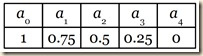Now recall the chart for evaluating conditionals.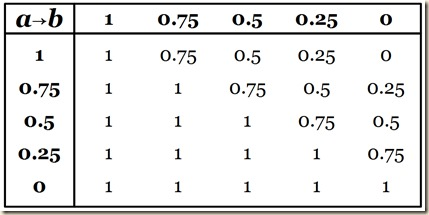Priest will note that to have a conditional where the antecedent is an and the consequent is an+1, the overall value of the conditional will always be 0.75. We can see this in chart [see the diagonal where all the values are 0.75]. [The idea might be that to say, ‘Jack is a child from any moment to the next’, where there are just 4 moments, is 0.75 true no matter which transition we consider. I am not sure, but I suppose the idea is that with each change some more of that truth value in comparison with the first state is lost, until the last status, when all of it is gone.]

aahas value 0.75 (=(1(1-0.75)); so does aa2 ; in fact, every conditional of the form an an+1 has the value 0.75.
(76)

[Priest then will show that no matter what level of acceptability we assign such situations leading to sorites paradoxes, the final inference in the sequence will be invalid. I do not grasp the reasoning here, so I will quote it below, and you can skip to it now. The idea seems to be the following. Recall the schema for how the sorites paradoxes work.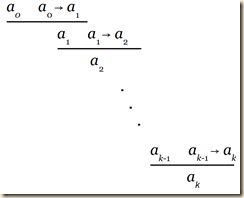We see that they use modus ponens throughout, since for each line we affirm the antecedent, then in the next line we begin with the inferred consequent, which now becomes the affirmed antecedent in the next conditional. Recall also our example, that since Jack is a child after just one second, he is therefore a child after no matter how many seconds. To see how Priest’s explanation works, it seems we need to note a couple things first. First recall the idea of validity. For an inference to be valid when values are fuzzy, we first assign an acceptable minimum value for a proposition to hold; then, for the inference to be valid, the conclusion must be at least that value, when the premises also meet at least that value. Now, suppose that we set the minimum value at 0.75. In our examples, we have for instance aa1. Here the values of the parts and the whole inference would be, 1 → 0.75, 0.75. Here everything is fine so far. Everything meets the minimum value. However, at some point, we need to get to a4, whose value is 0. Perhaps the idea here is that its conditional aa4 cannot be valid, because here one of the terms has the value 0, which means the minimum value would need to be 0, but that is too low for its validity to have any meaning. So on the one hand, in order for the modus ponens operation to work throughout the series of inferences, we need to set the minimum too low. If however on the other hand we set it at 1, none of the inferences will fulfill that minimum. This does not seem to be Priest’s reasoning exactly, so I probably have it wrong. Just go with what he says:]

What this tells us about the sorites paradox depends on the level of acceptability, ε, that is in force here. Suppose the context is one that imposes the highest level of acceptability; ε is 1. In this case, modus ponens is valid. For suppose that |a| = 1 and |a → b| = 1. Since |a → b| = 1, we must have |a| ≤|b|. It follows that |b| = 1. Thus the sorites argument is valid. In this case, though, each conditional premiss, having value 0.75, is unacceptable.

If, on the other hand, we set the level of acceptability lower than 1, then modus ponens turns out to be invalid. Suppose, for the sake of illustration, that ε is 0.75. As we have already seen, a, and aa1 both have value 0.75, but a2 has value 0.5, which is less than 0.75.

Either way you look at it, the argument fails. Either some of the | premisses aren’t acceptable; or, if they are, the conclusions don’t follow validly. Why are we taken in by sorites arguments so easily? Maybe because we confuse complete truth with near-complete truth. A failure to draw the distinction doesn’t make much difference normally. But if you do it again, and again, and again, ... it does.
(76-77)

Priest’s next point seems to be that even with fuzzy values, we still have not clarified the situation. [It seems the problem is the following. Consider the example of Jack growing up. In our fuzzy system, we know that at some point near the beginning he is not entirely a child (that is, the truth value of “Jack is a child” is not 1). But probably one millisecond after the first designation he is still fully a child. So we will need still to make an arbitrary division to know how long it takes before he becomes slightly less than a child. Now, the original motivation for using fuzzy values was that there was no clear-cut point along the development where Jack goes from simply being a child to simply not being one. But now, in order to go from a value of 1 to less than 1, we have the same problem. There does not seem to be an obvious place where this transition happens. Thus we have not solved the problem; we have merely relocated it to a place very near the beginning.]

That's one diagnosis of the problem. But with vagueness, nothing is straightforward. What was the problem about saying that ‘Jack is a child’ is simply true, until a particular point in time, when it becomes simply false? Just that there seems to be no such point. Any place one chooses to draw the line is completely arbitrary; it can be, at best, a matter of convention. But now, at what point in Jack’s growing up does he cease to be 100% a child; that is, at what point does ‘Jack is a child’ change from having the value of exactly 1, to a value below 1? Any place one chooses to draw this line would seem to be just as arbitrary as before. (This is sometimes called the problem of higher-order vagueness. ) If that is right, we haven’t really solved the most fundamental problem about vagueness: we have just relocated it.
(77)

[The following is quotation.]

Main Ideas of the Chapter

● Truth values are numbers between o and 1 (inclusive).
● |¬a | = 1 – |a|
● |a b| = Max(|a|, |b|)
● |a & b| = Min(|a|, |b|)
● If |a| ≤ |b|: |a→b| = 1
If |b| < |a|: |a→b| = 1 – (|a| – |b|) otherwise.
● A sentence is true in a situation just if its truth value is at least as great as the (contextually determined) level of acceptability.

(quoted from Priest, 77)

From:

Priest, Graham. Logic: A Very Short Introduction. Oxford: Oxford University, 2000.